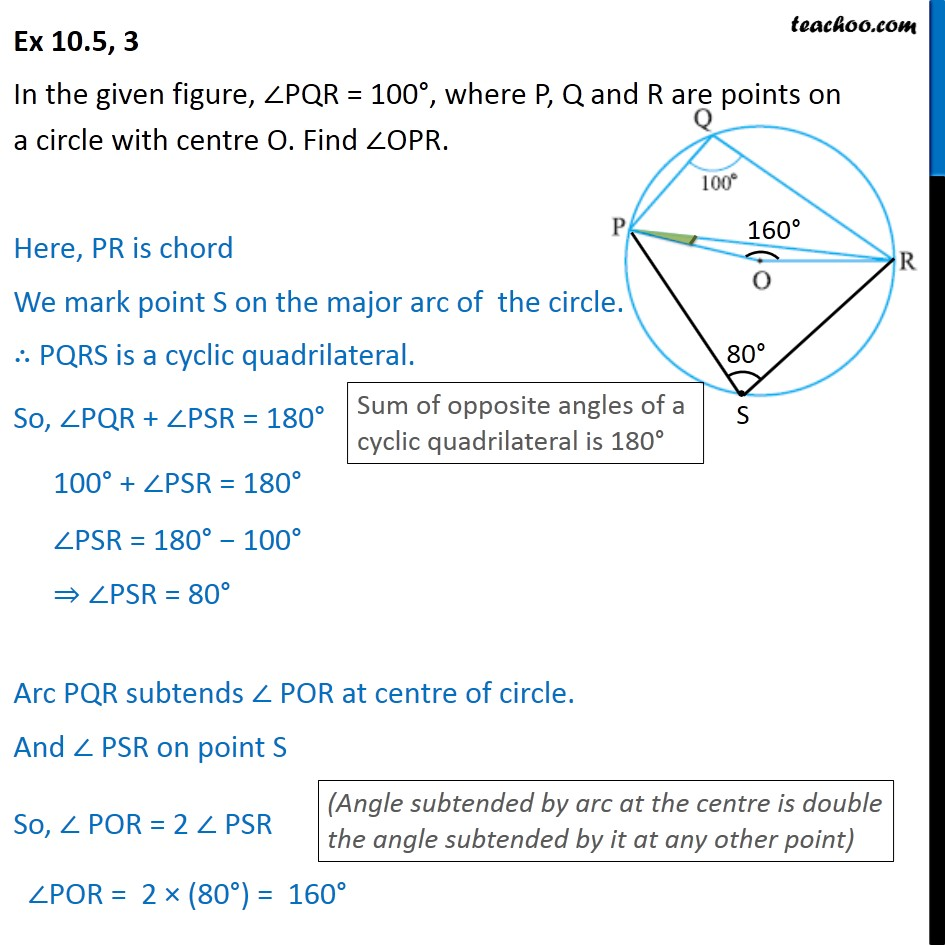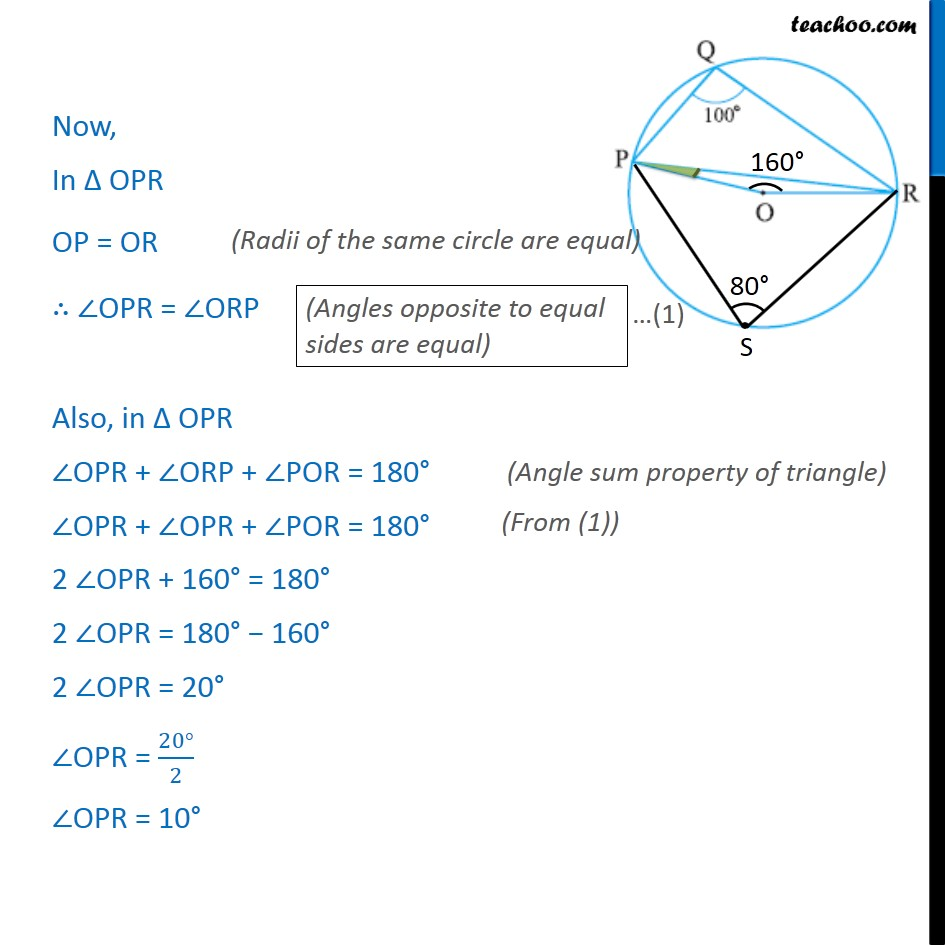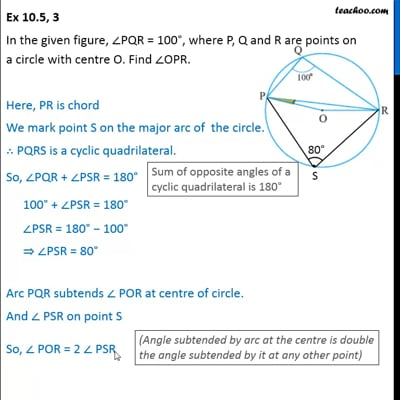Ex 10.5

Chapter 10 Class 9 Circles
Serial order wiseThis video is only available for Teachoo black users

Solve all your doubts with Teachoo Black (new monthly pack available now!)

### Transcript

Ex 10.5, 3 In the given figure, PQR = 100 , where P, Q and R are points on a circle with centre O. Find OPR. Here, PR is chord We mark point S on the major arc of the circle. PQRS is a cyclic quadrilateral. So, PQR + PSR = 180 100 + PSR = 180 PSR = 180 100 PSR = 80 Arc PQR subtends POR at centre of circle. And PSR on point S So, POR = 2 PSR POR = 2 (80 ) = 160 Now, In OPR OP = OR OPR = ORP Also, in OPR OPR + ORP + POR = 180 OPR + OPR + POR = 180 2 OPR + 160 = 180 2 OPR = 180 160 2 OPR = 20 OPR = (20 )/2 OPR = 10